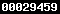# Second wave simulations

## Introduction

This page accompanies my article on COVID-19 second wave published in The Conversation, by providing simulation details.

The aim of this model is not to predict the type, size and timing of a potential “second wave” of the COVID-19 epidemic, but rather to provide an illustration of potential scenarios. As such, it is kept very simple.

## Model

The model used to produce the graphs is a basic SIR model with a time-dependent rate of spread. The population ofindividuals is split into four subpopulations, susceptible, infected, recoveredand dead. Initially, all are susceptible except a small proportion of individuals who are infected. There is no attempt to model latency or different stages in the disease.

The model is described in terms of four differential equations:whereis the time-dependent rate of spread,is the rate of removal, andis the proportion of deaths. Thus,is the length of the infectious period.

The time-dependent rate of spread is given by a combination of double logistic functions forming a pulse,wheredetermines how fast the rate changed in response to lockdown or release of lockdown,is the centre of the pulse, andis the width of the pulse.

The corresponding value of the current reproduction number is given byThe asymptomatic spread was modelled by assuming that only one in eight cases is symptomatic, i.e. the reporting rate is 12.5%.

For comparison with data, the number of infected individuals on any given day,was converted into reports by firstly dividing by the asymptomatic rate (8) and subsequently dividing by the infectious period.

## Parameter values

The infectious periodis a value widely published for COVID-19 data. The death probabilitywas estimated by comparing the output with the UK death data.

The values for the time-dependent rate are as follows:

The initial value ofis quite well established in the literature; it also agreed well with the initial growth in the UK , as shown in the graph below. The value ofduring the lockdown has also been attested by various methods for the UK data in late April and May. The increase to 1.2 was chosen to illustrate the danger of getting wrong the post-lockdown spread of the virus. The uncertainty of% is routinely reported for the values of.

The length of the pulses (50 days) reflect a combination of 7-14 days from the start of the increase in the rate to the detection of the increase by measuring, an implementation period for the future lockdown, and the period it takes for the lockdown measures to damp down the value of. 50 days just simply “looked good” by comparing the period with the “original” March-May 2020 lockdown.

The increase offor the autumn wave simulation was chosen to represent a high bound for the current estimate for SARS-CoV-2 ().

The probability that an infected individual dies,is equal to 0.019, i.e. 2%. This is broadly in agreement with data.

The initial number of all infected cases isand the simulations start on 5th March 2020. Other initial values were,,. Assuming only one-eighth of cases are symptomatic, this corresponds to 150 initially infected symptomatic individuals and to 19 reported cases (dividing further by the infectious period to convert infections to reports). The UK reported 48 cases on 5th March 2020.

## Results

Equations were solved in R using the odeSolve package. The parameters were chosen to represent UK data until 26th May 2020, as shown below, although no formal fitting procedure was carried out. As I intended to keep the article in The Conversation as generic as possible, I did not compare the UK data with the model output, but the comparison is shown below. Lines are model input (rate) and output (reported cases and deaths), points represent data (Johns Hopkins University, collected on 28th May 2020).The effective reproduction number, the number of reported cases and the total number of deaths as predicted by the model (solid line) compared to data for the UK (blue dots). The number of cases is estimated using the reporting rate of 12.5% and by dividing the number of infected individuals by the infectious period. No formal fitting was carried out. The period of the main lockdown is marked in grey; the start is 23rd March and the end is 23rd May as for England (Scotland, Northern Ireland and Wales implemented the lockdown for longer).The number of reported cases and the daily number of deaths comparison. The number of cases is estimated using the reporting rate of 12.5% and by dividing the number of infected individuals by the infectious period. No formal fitting was carried out. Red line corresponds to model and data in complete agreement.

## Caveats

The model is too simple to be able to predict the value ofat any given period as well as to fully account for the impact of the post-lockdown increase in. It cannot also be used to predict the number of cases or deaths in the future.

In the article, I did not want to go into details about simulations of deaths and did not show the comparison.

Thus, all the results in the article in The Conversation are for illustration purpose only.